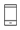Language:+86-576-87221128
Language:

# How can I calculate the over-steer or under-steer due to the anti-roll bar?

Update Time:2018/1/19
There's this great book called Fundamentals of Vehicle Dynamics by Thomas Gillespie. Chapter 6 of that book deals with cornering. The term he uses to describe cornering of a vehicle is steady state cornering. In the book, the equation for steering angle is given as follows,

Steer Angle = 57.3 L/R + K*(lateral acceleration)
where:
L = wheelbase
R = radius of curvature of curve
= understeer gradient (+ve if the car has understeer, -ve if oversteer, 0 if neutral).

An anti roll-bar is a part of automotive suspension that is used to reduce vehicle roll by stiffening up the suspension. So basically, it transfers the lateral load generated due to cornering.

Now, understeer gradient is the ratio of load on axle to cornering stiffness, i.e., W/C. When both ratios for front and rear axle are equal, it is termed as neutral steering (K=0). When the ratio of front is greater than the rear, it is termed as understeering (K>0). When the ratio of front is less than that of rear, it is termed as oversteering (K<0).

Since, lateral force (a) = W (V^2)/(C g R)

where,
g = acceleration due to gravity and
V= forward velocity,

we can predict whether the vehicle is going to understeer or oversteer by calculation and equating W/C ratios for front and rear and make necessary adjustment to the steering angle to compensate for the same.
Contact Person
Name:
Jessica
Tel:
0086-576-87221128
0086-13736677602
Email:
whatsapp:
86-13736677602